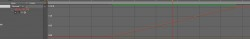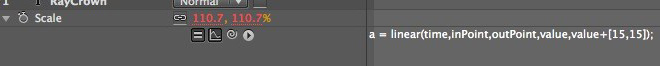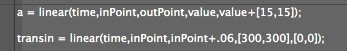# RayCrown讲inPoint和outPoint表达式

inPoint就是入点，outPoint就是出点啦。

inpoint和outpoint的值是数值，就是这个层的入点时间和出点时间。

linear(time,tmin,tmax,value,value+)的意思就是linear线性运动。

Number or Array linear(t, value1, value2) {t 是一个数, value1 和 value2 是一个数或数组}当t的范围从0到1时，返回一个从value1到value2的线性插值。当 t <= 0时返回value1，当 t >= 1时返回value2
Number or Array linear(t, tMin, tMax, value1, value2) {t, tMin和 tMax are 数, value1和value2 是数或数组} 当 t <= tmin时返回value1；当t >= tMax时，返回value2 ；当tMin < t < tMax 时， 返回 value1和value2 的线性联合。a = linear(time,inPoint,outPoint,value,value+[15,15]);
transin = linear(time,inPoint,inPoint+.06,[300,300],[0,0]);
transout = linear(time,outPoint-.06,outPoint,[0,0],[300,300]);
a+ transin + transout;